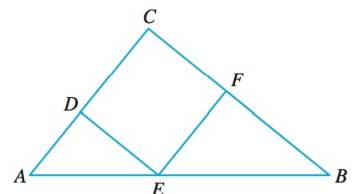Chapter A, Problem 15E### Algebra and Trigonometry (MindTap ...

4th Edition
James Stewart + 2 others
ISBN: 9781305071742

#### Solutions

Chapter
Section### Algebra and Trigonometry (MindTap ...

4th Edition
James Stewart + 2 others
ISBN: 9781305071742
Textbook Problem

# Proving Similarity In the figure CDEF is a rectangle. Prove that Δ ABC ∼ Δ AED ∼ Δ EBF .To determine

To prove:

That ΔABCΔAEDΔEBF in the given figure.

Explanation

Given:

The given figure is,

Figure (1)

Here, CDEF is a rectangle.

Approach:

Two triangles are similar if their vertices can be matched up so that corresponding angles are congruent. In this case corresponding sides are proportional.

If the two angles of the triangles are same then the third angle of the triangles have to be same, because the sum of angles in a triangle is 180°. Therefore, the triangles are similar by AA rule if two angles are same.

Proof:

It is given that CDEF is a rectangle.

So, C=EFB.

B is common in ΔABCandΔEBF.

Therefore, the two triangles are similar by AA rule.

Thus, ΔABCΔEBF

### Still sussing out bartleby?

Check out a sample textbook solution.

See a sample solution

#### The Solution to Your Study Problems

Bartleby provides explanations to thousands of textbook problems written by our experts, many with advanced degrees!

Get Started